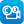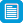# Search results

## Refine by topic

Main topic
Specific topic Related topic
Listed under:  Mathematics  >  Transformation (Geometry)  >  Congruence (Geometry)  >  Symmetry### Symmetry

An animated tutorial about types of polygons and their line symmetries, followed by an interactive quiz.### Axial Symmetry

An interactive applet in which students explore the effect of reflection in a variety of axes.### Sites2See: Space and Geometry for Primary

Selected links to online resources that can support the teaching and learning of geometry for primary-aged students.### reSolve: Reasoning With 3D Objects

This sequence of lessons explores symmetry and three dimensional objects. Students interpret and represent three-dimensional objects in two dimensions and apply their knowledge of transformations to show congruency or difference between objects. The lessons are outlined in detail including curriculum links, vocabulary, ...### reSolve: Authentic Problems: Expanded Square

This sequence of four lessons explores concepts around informal area and symmetry. Students design an 'expanded square' where approximately half the area of the original square is flipped to the outside. The lessons provide opportunities for students to devise and use methods to informally measure area, record their mathematical ...### Draw an egg

This teaching resource outlines an activity for students to draw an egg shape by hand, using a method that combines three ellipses. The resource outlines the materials required, provides instructions for drawing the curve, describes how the shape is composed of ellipses, and introduces some places where the egg shape is ...